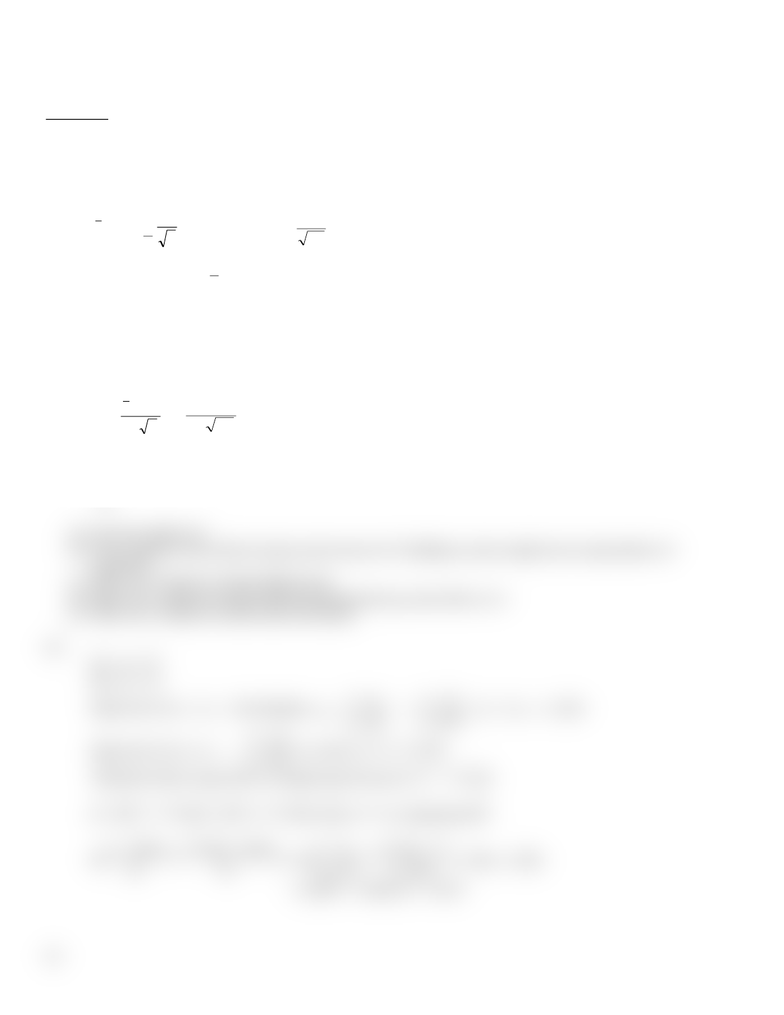Class Notes (1,100,000)
CA (640,000)
UTSC (30,000)
Lecture 1

# MGEB12H3 Lecture 1: assignment 1 solution (2015 winter)

Department
Economics for Management Studies
Course Code
MGEB12H3
Professor
Vinh Quan
Lecture
1

This preview shows half of the first page. to view the full 2 pages of the document.Department of Management, UTSC
MGEB12 Quantitative Methods in Economics II - Ass1 – Solution
Problem 1
(a) From output 95% 2-sided C.I:
72 – 2.4768 ≤ µ ≤ 72 + 2.4768 so 69.5232 ≤ µ ≤ 74.4768 so should not launch drink.
(b) For a 80% C.I. 100(1-α) = 80 get α = 0.2, d.f. = n – 1 = 25-1 = 24
n
s
tx
n
2
,1
α
±
25
0.6
0.72
1,.24
t
±
From
2/][
2
,11
α
α
=
nn
ttP
1.][
1,.2424
=
ttP
From t-dist table get t24,0.1 = 1.318
C.I. is 72 ± 1.816 or 70.4184 ≤ µ ≤ 73.5816
(c)
Ho: µ = 75
Ha: μ ≠ 75
Reject Ho if t0 ≤ -tα/2 or t0 ≥ tα/2
ns
x
t/
0
0
µ
=
=
25/6
7572
= -2.5, d.f. = n – 1 = 25-1 = 24
From
2/][
2/
α
α
=
ttP
→ P[t24 t24,.005] = 0.005 get t24,.005 = 2.797
Is -2.5 ≤ -2.797 or -2.5 2.797? No, so do not reject Ho. The mean rating is equal to 75.
Some sample recommendations:
(a) Increase sample size
(b) Take volunteers from other locations and not just in IC building to make sample more representative of
population.
(c) Make sure volunteers include different age
(d) Make sure volunteers include different background (eg, asian, black, etc.)
(e) Make sure volunteers include male and female.
(d)
Ho: µ≤ 75
Ha: μ > 75
Reject Ho if Z0 ≥ Zα , Test Statistic
n
x
Z/
0
0
σ
µ
=
=
, Zα = Z0.01 = 2.325
Reject Ho if Z0 Zα
2.325 or
x
77.325
Therefore fail to reject Ho or commit type II error if
x
< 77.325
β = P[
x
< 77.325] = P[
x
≤ 77.325] due to
x
is continuous RV
P[
xx
xExEx
σσ
][325.77][
] = P[
25/5
77325.77
/
n
x
a
σ
µ
] = P[z ≤ .325]
= (.6255 + .6293) /2 = .6274
(e)